Percentage Calculator

# Percentage calculator with step-by-step explanation

## Find the percentage of a number

To find the percentage of a number, you need to divide this number by 100 and multiply by the percentage, the value of which you need to find
To write a fraction, use the “/” sign, for example, 3/5 or -3/5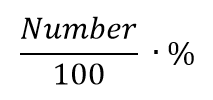## Find a number given its percent

To find a number by a known percentage, it is necessary to divide this number by percentage and multiply by 100.
To write a fraction, use the “/” sign, for example, 3/5 or -3/5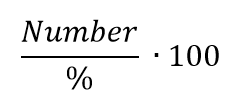## What percent one number is of another

To find what percent one number is of another, you need the number 1 divided by the number 2 and multiplied by 100
To write a fraction, use the “/” sign, for example, 3/5 or -3/5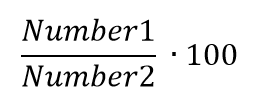## Add percent to a number

To add percent to a number, you must first find this percent of the number and add the result to the number
To write a fraction, use the “/” sign, for example, 3/5 or -3/5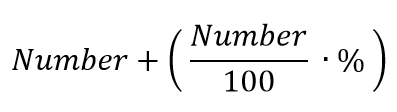## Subtract percent from a number

To subtract percent from a number, you must first find this percent of the number and subtract the result from the number
To write a fraction, use the “/” sign, for example, 3/5 or -3/5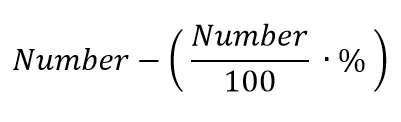Can we use your data to select ads for you? Our partners will collect information related to you and use cookies to personalize ads and evaluate their effectiveness. You can see a list of frequently used advertising technology providers.